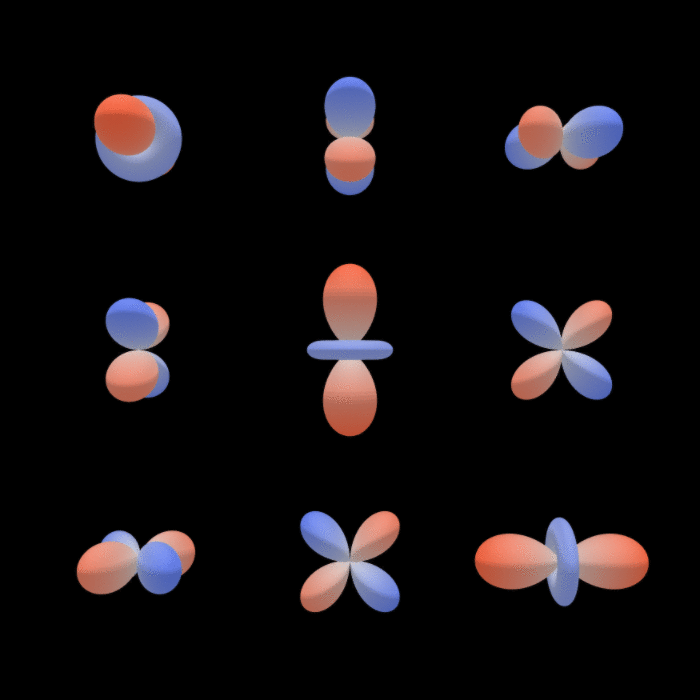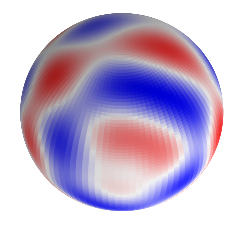# mariogeiger/se3cnn

Euclidean Neural Networks
Python Cuda C++
mariogeiger Merge pull request #44 from L-sky/point
`Kernel: normalization`
Latest commit 994b5a3 Nov 27, 2019
Type Name Latest commit message Commit time
Failed to load latest commit information.examples Oct 2, 2019se3cnn Nov 26, 2019src/real_spherical_harmonics Nov 14, 2019tests Nov 26, 2019.gitignore Jul 17, 2019LICENSE Jul 23, 2019README.md Nov 20, 2019setup.py Nov 13, 2019

# SE3CNN

The group SE(3) is the group of 3 dimensional rotations and translations. This library aims to create SE(3) equivariant convolutional neural networks.## Image and Point

The code is separated in two parts:

• `image` for volumetric data 
• `point` for point cloud data 

## Example

### Image

```import torch
from se3cnn.image.convolution import SE3Convolution

size = 32  # space size

scalar_field = torch.randn(1, 1, size, size, size)  # [batch, _, x, y, z]

Rs_in = [(1, 0)]  # 1 scalar field
Rs_out = [(1, 1)]  # 1 vector field
conv = SE3Convolution(Rs_in, Rs_out, size=5)
# conv.weight.size() ==  (2 radial degrees of freedom)

vector_field = conv(scalar_field)  # [batch, vector component, x, y, z]

# vector_field.size() == [1, 3, 28, 28, 28]```

### Point

```from functools import partial
import torch
from se3cnn.point.kernel import Kernel
from se3cnn.point.operations import Convolution
from se3cnn.util.plot import plot_sh_signal
import matplotlib.pyplot as plt

# Radial model:  R -> R^d
# Projection on cos^2 basis functions followed by a fully connected network

# kernel: composed on a radial part that contains the learned parameters
#  and an angular part given by the spherical hamonics and the Clebsch-Gordan coefficients

# Use the kernel to define a convolution operation
C = partial(Convolution, K)

Rs_in = [(1, 0)]  # one scalar
Rs_out = [(1, l) for l in range(10)]
conv = C(Rs_in, Rs_out)

n = 3  # number of points
features = torch.ones(1, n, 1)
geometry = torch.randn(1, n, 3)

features = conv(features, geometry)

plt.figure(figsize=(4, 4))
plot_sh_signal(features[:, 0], n=50)
plt.gca().view_init(azim=0, elev=45)```## Hierarchy

• `se3cnn` contains the library
• `se3cnn/SO3.py` defines all the needed mathematical functions
• `se3cnn/image` contains the code specific to voxels
• `se3cnn/point` contains the code specific to points
• `se3cnn/non_linearities` non linearities working for both point and voxel code
• `examples` simple scripts and experiments

## Installation

1. install pytorch
2. `pip install git+https://github.com/AMLab-Amsterdam/lie_learn`
3. `pip install git+https://github.com/mariogeiger/se3cnn`

## Usage

Install with

``````python setup.py install
``````

## Citing``````@misc{mario_geiger_2019_3348277,
author       = {Mario Geiger and
Tess Smidt and
Wouter Boomsma and
Maurice Weiler and
Michał Tyszkiewicz and
Jes Frellsen and
Benjamin K. Miller},
title        = {mariogeiger/se3cnn: Point cloud support},
month        = jul,
year         = 2019,
doi          = {10.5281/zenodo.3348277},
url          = {https://doi.org/10.5281/zenodo.3348277}
}
``````
You can’t perform that action at this time.